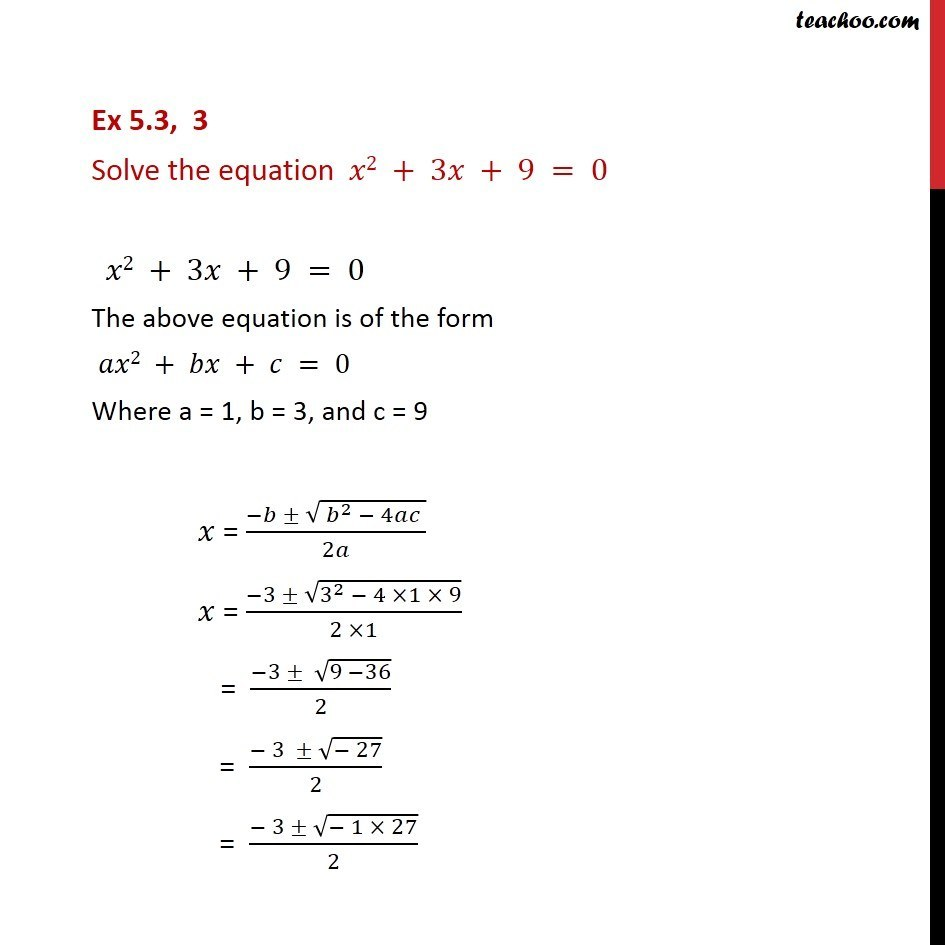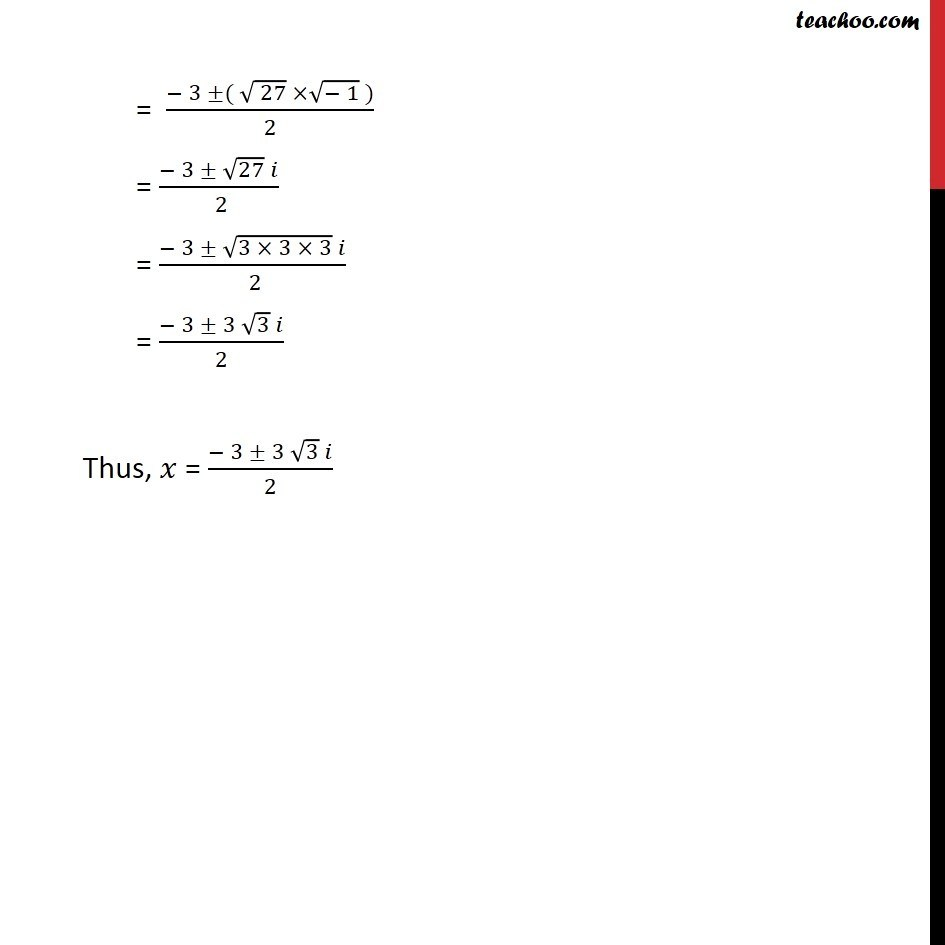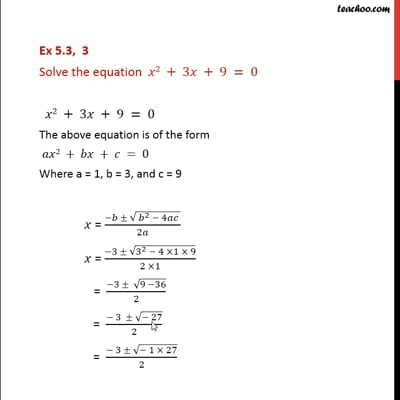Ex 5.3

Chapter 5 Class 11 Complex Numbers
Serial order wiseThis video is only available for Teachoo black users

Solve all your doubts with Teachoo Black (new monthly pack available now!)

### Transcript

Ex 5.3, 3 Solve the equation 2 + 3 + 9 = 0 2 + 3 + 9 = 0 The above equation is of the form 2 + + = 0 Where a = 1, b = 3, and c = 9 = ( ( ^2 4 ))/2 = ( 3 (3^2 4 1 9))/(2 1) = ( 3 (9 36))/2 = ( 3 ( 27))/2 = ( 3 ( 1 27))/2 = ( 3 ( ( 27) ( 1) ))/2 = ( 3 27 )/( 2) = ( 3 (3 3 3) )/( 2) = ( 3 3 3 )/2 Thus, = ( 3 3 3 )/2